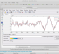## Combining two wave generation formulas not working as expected (MATLAB/OCTAVE)Started by 2 years ago4 replieslatest reply 2 years ago131 views

I'm trying to convert Mathematica sawtooth wave calculation formula to Octave/Matlab language. Here are the original formulas. My Desmos take is here

I've got formulas for triangle wave and square wave working but, sawtooth won't work (when done in either way like showed in Desmos sheet (two last formulas there)).

Here's my Octave code (active saw calculation is simplified version of the above commented equation):

function saw = saw_wave_trig(fs, f0, s, m, w)
% fs = sample rate
% f0 = fundamental frequency
% s  = 'sharpness'
% w  = 'width'
% m  = 'tooth direction'
% example: saw = saw_wave_trig(44100, 440, 0.03, -2, 1);
t = 0:1/fs:1;
##saw = m .* ((1.+ triangle_wave_trig(fs, (2*(f0/w)+1)/4, s, w) .* ...
##              square_wave_trig(fs, f0/2, s, w))./2) ...
##     .- sign(m);
##saw =  m .* ...
##            (1 .+ ...
##                  ((1.-(2.*acos((1-s) .* sin((2*pi*((2*(f0/w)+1)/4)).*t')))./pi) .* ...
##                       (2 .* atan(sin((2*pi*(f0/w/2)) .* t')./s))./pi)) ./ ...
##                         2 .- sign(m);
saw = (4.*asin((s-1).*sin((pi*t'.*(2*f0+w))./ ...
(2*w))).*acot(s*csc((pi*f0.*t')./w)))./pi^2-sign(m)-1;
audiowrite('test_saw_trig_wave.wav',saw, fs);
plot(t, saw);


which (the commented calculations as well) produces kind of morph from negative side triangle wave to correct looking sawtooth wave, as seen in attached plot (Audacity used):

So, as my knowledge in math and Octave/Matlab is quite limited, there sure is some basics done wrong in my Octave/Matlab code. Maybe the multiplication of triangle and square needs to be done differently?

Any help in solving this is appreciated.

P.S. This is part of experiment I'm doing with various wave generation methods out there!

[ - ]It works, (In your code, I noticed the wrong use of "t" in your calculations. I tried to change your code, you may check below )function saw = saw_wave_trig(fs, f0, s, m, w)
% fs = sample rate
% f0 = fundamental frequency
% s  = 'sharpness'
% w  = 'width'
% m  = 'tooth direction'

% example: saw = saw_wave_trig(44100, 440, 0.03, -2, 1);

t = 0:1/fs:4;
##saw = m .* ((1.+ triangle_wave_trig(fs, (2*(f0/w)+1)/4, s, w) .* ...
##              square_wave_trig(fs, f0/2, s, w))./2) ...
##     .- sign(m);

saw =  m * ...
(1 + ((1.-(2*acos((1-s) * sin((2*pi*((2*(t/w)+1)/4)))))./pi) .* ...
(2 .* atan(sin((pi*(t/w)) )/s))./pi)) / ...
2 - sign(m);

##saw = (4.*asin((s-1).*sin((pi*t'.*(2*f0+w))./ ...
##        (2*w))).*acot(s*csc((pi*f0.*t')./w)))./pi^2-sign(m)-1;
plot(t, saw);
audiowrite('test_saw_trig_wave.wav',saw, 44100);
end

saw = saw_wave_trig(44100, 440, 0.03, -2, 1)

[ - ]Thanks, it could work that way as it does in Desmos sheet but, I need to specify the fundamental frequency f0 at some point in both sections (triangle and square) to get proper sawtooth wave form.

Here are those triangle_wave_trig() and square_wave_trig() functions (f1() and f2() in Desmos sheet) which of both works correctly:

function tri = triangle_wave_trig(fs, f0, s, w) % fs = sample rate % f0 = fundamental frequency % s  = 'sharpness' % w  = 'width' % example: tri = triangle_wave_trig(44100, 440, 0.03, 1);    t = 0:1/fs:1;   tri = 1 .- (2 .* acos((1 - s) .* sin((2 * pi * (f0 / w)) .* t')) ./ pi);     audiowrite('test_triangle_wave.wav',tri, fs);   plot(t, tri);

function sqw = square_wave_trig(fs, f0, s, w)% fs = sample rate% f0 = fundamental frequency% s  = 'sharpness'% w  = 'width'% example: sqw = square_wave_trig(44100, 440, 0.03, 1);  t = 0:1/fs:1;  sqw = 2.0 .* (atan(sin((2 * pi * (f0 / w)) .* t') ./ s) ./ pi);  audiowrite('test_square_wave_trig.wav',sqw, fs);  plot(t, sqw);  hold on;  plot(t, square(2*pi*f0*t'));

sqw = square_wave_trig(44100, 440, 0.03, 1); looks like this:and generated wave file looks OK.

[ - ]Yes, this is what you want I think  (I plot f0=5 case to show clearly that it works);function saw = saw_wave_trig(fs, f0, s, m, w)
% fs = sample rate
% f0 = fundamental frequency
% s  = 'sharpness'
% w  = 'width'
% m  = 'tooth direction'

% example: saw = saw_wave_trig(44100, 440, 0.03, -2, 1);

t = 0:1/fs:4;
##saw = m .* ((1.+ triangle_wave_trig(fs, (2*(f0/w)+1)/4, s, w) .* ...
##              square_wave_trig(fs, f0/2, s, w))./2) ...
##     .- sign(m);

saw =  m .* ...
(1 .+ ...
((1.-(2.*acos((1-s) .* sin((2*pi*((2*(t*f0/w)+1)/4)))))./pi) .* ...
(2 .* atan(sin((2*pi*(t*f0/w/2)) )./s))./pi)) ./ ...
2 .- sign(m);

##saw = (4.*asin((s-1).*sin((pi*t'.*(2*f0+w))./ ...
##        (2*w))).*acot(s*csc((pi*f0.*t')./w)))./pi^2-sign(m)-1;

audiowrite('test_saw_trig_wave.wav',saw, fs);
plot(t, saw);
end

saw = saw_wave_trig(44100, 5, 0.03, -2, 1);


[ - ]